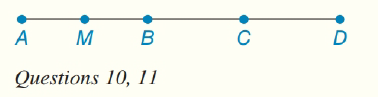Chapter 1.CT, Problem 11CT### Elementary Geometry for College St...

6th Edition
Daniel C. Alexander + 1 other
ISBN: 9781285195698

#### Solutions

Chapter
Section### Elementary Geometry for College St...

6th Edition
Daniel C. Alexander + 1 other
ISBN: 9781285195698
Textbook Problem
1 views

# In the figure, A B = x , B D = x + 5 , and A D = 27 .Find: a) x _______ b) B D _________To determine

(a)

To find:

The value of x.

Explanation

Given:

The given figure is shown below.

Approach:

According to Segment-Addition Postulate, if two points A, B are given on a line segment, then third point C lies on the line segment if and only if,

AC+CB=AB

To determine

(b)

To find:

The value of BD.

### Still sussing out bartleby?

Check out a sample textbook solution.

See a sample solution

#### The Solution to Your Study Problems

Bartleby provides explanations to thousands of textbook problems written by our experts, many with advanced degrees!

Get Started

#### The slope of the tangent line to r = cos θ at is:

Study Guide for Stewart's Multivariable Calculus, 8th

#### Subtract 7516278 .

Mathematics For Machine Technology

#### What can a researcher do if the treatment administered during the first B?

Research Methods for the Behavioral Sciences (MindTap Course List)